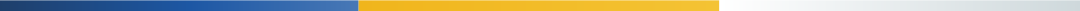# 夫妻离婚房产怎样分割？法官给出了一个计算公式！

#### ——

2019-08-26 15:54:542007年风和日丽的一天

？？？？（本金180000元，利息45419元）👇
👇

a=首付款金额
b=按揭贷款金额
c=贷款利息
d=离婚时房产价值（可由双方协商确认，或由专业机构评估定价）
e=夫妻共同还贷本息金额（可在贷款银行中查询记录）
☀第二，计算夫妻共同还贷部分占房价款的比例y
y=e÷（a+b+c）
☀第三，计算找补金额za=首付款金额=122800元
b=按揭贷款金额=180000元
c=贷款利息=45419元
d=离婚时房产价值=580000元
e=夫妻共同还贷本息金额=203360.55元
y=e÷（a+b+c）=203360.55÷（122800+180000+45419）=58.4%

z=d×y÷2=580000×58.4%÷2=169360元；

z=d-d×y÷2
=580000-580000×58.4%÷2=410640元

《中华人民共和国婚姻法》

（一）工资、奖金；

（二）生产、经营的收益；

（三）知识产权的收益；

（四）继承或赠与所得的财产，但本法第十八条第三项规定的除外；

（五）其他应当归共同所有的财产。